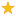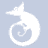quizzes  Forums# Acids and Bases

created by Vicky8110… on 7 Apr 14, enabled by LouVery hard level (23% of success)    12 questions - 1 010 players
You must base on chemistry.
They are difficult.1
Which one of these statements about strong acids is true?

2
Determine the pH of a KOH solution made by mixing 0. 251g KOH with enough water to make 1. 00 x 10² mL of solution.

3
What is the pH of 10. 0 mL of 0. 0020M HCL?

4
Calculate the pH of a 0. 10M HCN solution that is 0. 0070% ionized.

5
What is the pH of a 0. 0055M HA (weak acid) solution that is 8. 2% ionized?

6
Calculate the pH of a 1. 6M KOH solution.

7
Calculate the pH of 6. 71 x 10¯² M NAOH solution.

8
Calculate the pH of 2. 6 x 10¯² M KOH.

9
What is the pH of a solution prepared by mixing 10. 0mL of a strong acid solution with pH=2 and 10. 0mL of a strong acid solution with pH=6. 00?

10
When comparing acid strength of binary acids HX, as X varies within a particular group of the period table, which one of these factors dominates in affecting the acid strength?

11
Hard water deposits (calcium carbonate) have built up around your bathroon sink. Which one of these substances would be most effective in dissolving the deposits?

12
Determine the pH of a KOH solution made by mixing 0. 251g KOH with enough water to make 1. 00 x 10² mL of solution.